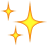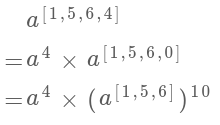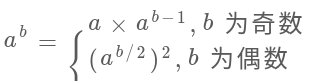• java中直接写double x=Math.pow(a,b);即可，不需要额外地导入包。
java中直接写double x=Math.pow(a,b);即可，不需要额外地导入包。
展开全文java
• Super Pow题目递归python代码 题目 Your task is to calculate ab mod 1337 where a is a positive integer and b is an extremely large positive integer given in the form of an array. Example 1: Input: a = 2...


Super Pow
题目递归python代码

题目
Your task is to calculate ab mod 1337 where a is a positive integer and b is an extremely large positive integer given in the form of an array.
Example 1:
Input: a = 2, b =  Output: 8
Example 2:
Input: a = 2, b = [1,0] Output: 1024
递归
大多数的问题都不是一眼直接看出来的，一般都要从基本的方法当中找规律。 1、处理数组指数 数组够长的话是不能直接转化成int的，所以要另想他法。 通常a^b = aa^(b-1)，在数组中可类似计算。 2、求模 公式：（ab）%MOD = (a%MOD)(b%MOD)%MOD 证明： a = Ak +B；b = Ck + D ab = ACk^2 + ADk + BCk +BD a % k = B；b % k = D BD % k = (a % k)(b % k) % k
python代码
class Solution(object):
def superPow(self, a, b):
"""
:type a: int
:type b: List[int]
:rtype: int
"""
if not b:
return 1
k = b.pop()
p1 = self.myPow(a,k)
p2 = self.myPow(self.superPow(a,b),10)
MOD = 1337
return (p1%MOD)*(p2%MOD)%MOD

def myPow(self,a,k):
if not k:
return 1
MOD = 1337
a %= MOD
res = 1
for i in range(k):
res *= a
res %= MOD
return res

展开全文• 那就不妨继续读下去，我来教大家一个更高效的求幂运算 让我们有请——快速幂！！！ 快速幂逻辑讲解 举例 假设我们求3的5次方，即底数是3，指数是5 首先，我们将5转化为二进制，即101 其次，按位将二进制的指数拆解，...


别再用pow函数做算法题了
引文快速幂逻辑讲解举例总结
快速幂代码讲解最后

引文
众所周知，pow函数是最简单，也是效率最低的求幂函数。如果不对其进行相应的优化，很多算法题光是从运行时间上就无法通过。
我相信大家不是不想用更高效的求幂算法，但苦于不知道。那就不妨继续读下去，我来教大家一个更高效的求幂运算
让我们有请——快速幂！！！
快速幂逻辑讲解
举例
假设我们求3的5次方，即底数是3，指数是5
首先，我们将5转化为二进制，即101
其次，按位将二进制的指数拆解，写成101=20+22
所以35=32的0次方+2的2次方=31*34
总结
也就是说快速幂就是将指数转化为二进制，然后观察哪个位是1，个位是1就乘上1个底数，十位是1就乘上2个底数依次类推
所以对于35，将5转化为二进制101，那么最后的结果就是1（20）个3乘上4（22）个3
快速幂代码讲解
先上代码：
int Pow(int a,int b)
{
int ans=1,base=a;
while(b)
{
if(b&1) ans*=base;
base*=base;
b>>=1;
}
return ans;
}

还是拿35举例
第6行中的if(b&1)是按位相与的意思，不知道&即按位相与是什么意思的同学可以先跳转到下面这篇文章学习一下再回来继续理解
还没搞懂&与&&在c语言中的区别？快点进来看看！
b&1j就是看最低位是否为1，如果是1就进行乘上对应权重个底数
而第7行base*=base;的意思就是使base与对应二进制的位数相匹配，即从右往左第一位是1个a，第二位是2个a，第3位是4个a
最后，第八行b>>=1;就是将指数b的二进制数向右移动1位，类似于求各位数字时n=n/10的操作
最后
如果想更为详细地了解快速幂，可以参考这篇文章 https://blog.csdn.net/iwts_24/article/details/79780596
展开全文算法
• 点击上方蓝字设为星标东哥带你搞定算法~今天来聊一道与数学运算有关的算法题目，LeetCode 372 题 Super Pow，让你进行巨大的幂运算，然后求余数。intsuperPow(...


点击上方蓝字设为星标东哥带你搞定算法~今天来聊一道与数学运算有关的算法题目，LeetCode 372 题 Super Pow，让你进行巨大的幂运算，然后求余数。
int superPow(int a, vector<int>& b);

要求你的算法返回幂运算a^b的计算结果与 1337 取模（mod，也就是余数）后的结果。就是你先得计算幂a^b，但是这个b会非常大，所以b是用数组的形式表示的。
这个算法其实就是广泛应用于离散数学的模幂算法，至于为什么要对 1337 求模我们不管，单就这道题可以有三个难点：
一是如何处理用数组表示的指数，现在b是一个数组，也就是说b可以非常大，没办法直接转成整型，否则可能溢出。你怎么把这个数组作为指数，进行运算呢？
二是如何得到求模之后的结果？按道理，起码应该先把幂运算结果算出来，然后做% 1337这个运算。但问题是，指数运算你懂得，真实结果肯定会大得吓人，也就是说，算出来真实结果也没办法表示，早都溢出报错了。
三是如何高效进行幂运算，进行幂运算也是有算法技巧的，如果你不了解这个算法，后文会讲解。
那么对于这几个问题，我们分开思考，逐个击破。
如何处理数组指数
首先明确问题：现在b是一个数组，不能表示成整型，而且数组的特点是随机访问，删除最后一个元素比较高效。
不考虑求模的要求，以b = [1,5,6,4]来举例，结合指数运算的法则，我们可以发现这样的一个规律：看到这，我们的老读者肯定已经敏感地意识到了，这就是递归的标志呀！因为问题的规模缩小了：
    superPow(a, [1,5,6,4])
=>  superPow(a, [1,5,6])

那么，发现了这个规律，我们可以先简单翻译出代码框架：
// 计算 a 的 k 次方的结果
// 后文我们会手动实现
int mypow(int a, int k);

int superPow(int a, vector<int>& b) {
// 递归的 base case
if (b.empty()) return 1;
// 取出最后一个数
int last = b.back();
b.pop_back();
// 将原问题化简，缩小规模递归求解
int part1 = mypow(a, last);
int part2 = mypow(superPow(a, b), 10);
// 合并出结果
return part1 * part2;
}

到这里，应该都不难理解吧！我们已经解决了b是一个数组的问题，现在来看看如何处理 mod，避免结果太大而导致的整型溢出。
如何处理 mod 运算
首先明确问题：由于计算机的编码方式，形如(a * b) % base这样的运算，乘法的结果可能导致溢出，我们希望找到一种技巧，能够化简这种表达式，避免溢出同时得到结果。
比如在二分查找中，我们求中点索引时用(l+r)/2转化成l+(r-l)/2，避免溢出的同时得到正确的结果。
那么，说一个关于模运算的技巧吧，毕竟模运算在算法中比较常见：
(a*b)%k = (a%k)(b%k)%k
证明很简单，假设：
a=Ak+B；b=Ck+D
其中 A,B,C,D 是任意常数，那么：
ab = ACk^2+ADk+BCk+BD
ab%k = BD%k
又因为：
a%k = B；b%k = D
所以：
(a%k)(b%k)%k = BD%k
综上，就可以得到我们化简求模的等式了。
换句话说，对乘法的结果求模，等价于先对每个因子都求模，然后对因子相乘的结果再求模。
那么扩展到这道题，求一个数的幂不就是对这个数连乘么？所以说只要简单扩展刚才的思路，即可给幂运算求模：
int base = 1337;
// 计算 a 的 k 次方然后与 base 求模的结果
int mypow(int a, int k) {
// 对因子求模
a %= base;
int res = 1;
for (int _ = 0; _ < k; _++) {
// 这里有乘法，是潜在的溢出点
res *= a;
// 对乘法结果求模
res %= base;
}
return res;
}

int superPow(int a, vector<int>& b) {
if (b.empty()) return 1;
int last = b.back();
b.pop_back();

int part1 = mypow(a, last);
int part2 = mypow(superPow(a, b), 10);
// 每次乘法都要求模
return (part1 * part2) % base;
}

你看，先对因子a求模，然后每次都对乘法结果res求模，这样可以保证res *= a这句代码执行时两个因子都是小于base的，也就一定不会造成溢出，同时结果也是正确的。
至此，这个问题就已经完全解决了，已经可以通过 LeetCode 的判题系统了。
但是有的读者可能会问，这个求幂的算法就这么简单吗，直接一个 for 循环累乘就行了？复杂度会不会比较高，有没有更高效的算法呢？
有更高效的算法的，但是单就这道题来说，已经足够了。
因为你想想，调用mypow函数传入的k最多有多大？k不过是b数组中的一个数，也就是在 0 到 9 之间，所以可以说这里每次调用mypow的时间复杂度就是 O(1)。整个算法的时间复杂度是 O(N)，N 为b的长度。
但是既然说到幂运算了，不妨顺带说一下如何高效计算幂运算吧。
如何高效求幂
快速求幂的算法不止一个，就说一个我们应该掌握的基本思路吧。利用幂运算的性质，我们可以写出这样一个递归式：这个思想肯定比直接用 for 循环求幂要高效，因为有机会直接把问题规模（b的大小）直接减小一半，该算法的复杂度肯定是 log 级了。
那么就可以修改之前的mypow函数，翻译这个递归公式，再加上求模的运算：
int base = 1337;

int mypow(int a, int k) {
if (k == 0) return 1;
a %= base;

if (k % 2 == 1) {
// k 是奇数
return (a * mypow(a, k - 1)) % base;
} else {
// k 是偶数
int sub = mypow(a, k / 2);
return (sub * sub) % base;
}
}

这个递归解法很好理解对吧，如果改写成迭代写法，那就是大名鼎鼎的快速幂算法。至于如何改成迭代，很巧妙，这里推荐一位大佬的文章 让技术一瓜共食：快速幂算法。
虽然对于题目，这个优化没有啥特别明显的效率提升，但是这个求幂算法已经升级了，以后如果别人让你写幂算法，起码要写出这个算法。
至此，Super Pow 就算完全解决了，包括了递归思想以及处理模运算、幂运算的技巧，可以说这个题目还是挺有意思的，你有什么有趣的题目，可以留言分享一下。
历史文章：
回溯算法团灭排列/组合/子集问题
加密算法的前世今生
这个问题不简单：寻找缺失元素展开全文• “**”运算这个“**”比较简单，就是标题中的python的幂运算了 2 ** 012 ** 122 ** 1010242 ** 201048576第一操作数为底数，第二个操作数则为指数...“”运算除法运算符是“”，这个人人皆知道，但是这个二元运算符...
• //幂运算** console.log(2**10) //1024 console.log(Math.pow(2,10)) //1024
• In numpy, I have done the following: result = numpy.sqrt(numpy.sum(numpy.pow(some_vector, 2))) And in pure python something like this would be expected: result = math.sqrt(math.pow(A, 2) + math.pow(B...
• In numpy, I have done the following: result = numpy.sqrt(numpy.sum(numpy.pow(some_vector, 2))) And in pure python something like this would be expected: result = math.sqrt(math.pow(A, 2) + math.pow(B...
• Problem：实现幂运算pow(x,n) 设形式为pow(x,n)= x^n，则有一下分支： 当x==0时，返回0 当n==0时，返回1 当n<0时，（此时需要注意，不能直接将n = -n，因为最小负数变为相反数之后会超过int的最大范围）...
• 实现浮点类型的幂运算，函数原型为: double pow(double x, int n) 下面介绍一下解决该问题的几种方法以及要注意的地方： 1)最直观容易想到的方法就是用递归方法求n个x的乘积，注意考虑n的正负号，时间...c++ leetcode
• 幂运算，简单的方法snag然很好实现，直接循环相乘就可以了，但是这里应该不是那种那么简单，我的做法使用到了一点递归： 1 class Solution { 2 public: 3 double myPow(double x, int n) 4 { 5 if(n == ...
• pow(double x,double y) 计算x的y次，返回一个double型结果 包含于math.h头文件 参考：C++ - pow函数
• 幂运算： package operator; public class Demo02 { public static void main(String[] args) { //幂运算 2^3 运算利用工具类来操作 double pow=Math.pow(2, 3); System.out.println(pow); } } 位运算左移...java
• 幂运算（快速幂）暴力幂运算Math类下的pow(double a, double b)快速幂（涉及位运算）测试 暴力幂运算 public static long pow1(int a, int b){// 求 a^b long ans = 1; for (int i = 0; i < b; i++) { ans *= ...java
• 函数一讲中的pow方法实现 今天啊，看到了函数一讲，练习题中，有这么一个问题。 题：写一个函数 pow(x,n)，返回 x 的 n 次方。换句话说，将 x 与自身相乘 n 次，返回最终结果。 pow(3, 2) = 3 * 3 = 9 pow(3, 3) = 3...js javascript es6
• 问题描述：Implement pow(x, n).实现浮点类型的幂运算。问题求解：方法一：递归，O（n）解法。class Solution { public: double myPow(double x, int n) { if(n==0) return 1.0; if(n) { if
• Implement pow(x,n). Subscribeto see which companies asked this question 解法1：最简单的即是n个x直接相乘，毫无疑问会超时Time Limit Exceeded class Solution { public: double myPow(double x,...
• 在Swift中想求幂运算、有系统函数pow(x,y)，含义为：求x的y次幂 public func pow(_ x: Decimal, _ y: Int) -> Decimal Decimal根据系统文档表示为 表示以10为基数的数的结构体，就是十进制的表示方法，可以...
• 我们最常用的幂运算是，使用一个循环语句来控制循环乘或者直接使用库函数pow(int a,int n)来实现幂运算。 使用循环语句来进行幂运算，时间复杂度是O(n) 再进行网上查询了解到pow库函数的时间复杂度会比O(n)还大 了解...算法 数据结构
• 实现 pow(x,n)，即计算x的n次函数。 示例 1: 输入: 2.00000, 10 输出: 1024.00000 示例 2: 输入: 2.00000, -2 输出: 0.25000 解释: 2-2 = 1/22 = 1/4 = 0.25 解题报告 核心就是减少重复计算。例如 410=(45)24^{10}...
• // return pow(x,n/2)*pow(x,n/2) 会影响程序效率 } else { return pow( x, n-1) * x; } } // 判断奇偶 public static boolean isEven(int n) { if (n % 2 == 0) { return true; } else { ...java
• ## C++幂运算

千次阅读 2020-01-11 14:51:59
头文件#include<math.h> 使用函数pow(x,y)，就可以求x的y次幂 实在忘了可以用循环for(int i = 0;i < y;i++)x * x;来进行幂运算 注：^并不是求幂运算符，而是按位异或运算符 ...c++
• 在我们求幂运算的时候，一般的朴素算法也就是o(n)的时间复杂度 //o(n)的时间复杂度来求幂运算 for(int i = 1; i < n; i++) { ans = ans * x; } 但是，在我们求的幂方很大的时候就会浪费时间，这个时候就需要...算法
• 问题原始链接https://leetcode.com/problems/powx-n 实现 Pow(x, n)。 思路：二分法。令 half = Pow(x, n/2)，如果 n 为偶数，则结果为 half*half，如果n为奇数，则结果为 half*half*x。 public cl......